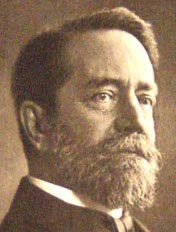## Saturday, October 08, 2005

### Mathematical Models

"Backwards" might mean, from a "5d understanding" to a three dimensional fabrication. You had to understand how the 5d world is explained here, before judgement is cast.

While I would like nothing more then to cater to the struggles of good professors, to the aims they had set for themselves, it has come to me, that mathematic modelling is culminative. Where does this point too?

If indeed a good understanding has been established, regardless of string theory or any association for that matter, this stand alone item on Langlands or what ever one might associated it too, would of itself, painted it's own picture and further associations, from a basis and exposition of that mathemtical derivative.

It does not have to be associated it either to historical figures (like Plato), other then the ones we trace to the orignation and factors that brought other areas of mathematics together. This should be readily available, by doing searches which I did. Although I sahl say there is much that needs to be resolved, in our determinations of truth. I am working on understanding this here.

But then to take it further, I wanted to illustrate this point just a little more on imaging.

Thomas Banchoff has set this straight in terms of modelling in computerization and what it can do for us in 5D expressions? While in the Wunderkammern of this site such model although concretize in form have relative associations in computerization value much like the model of the Klein bottle exemplified of itself in acme products.

x = cos(u)*(cos(u/2)*(sqrt_2+cos(v))+(sin(u/2)*sin(v)*cos(v)))

y = sin(u)*(cos(u/2)*(sqrt_2+cos(v))+(sin(u/2)*sin(v)*cos(v)))

z = -1*sin(u/2)*(sqrt_2+cos(v))+cos(u/2)*sin(v)*cos(v)

or in polynomial form:

Yep, no doubt about it: Your Acme's Klein Bottle is a real Riemannian manifold, just waiting for you to define a Euclidean metric at every point.Felix Klein
When Klein became a Professor in Leipzig in 1880, he immediately started to acquire mathematical models and establish a model collection. Klein was a geometer and used these plaster models in his university lectures. Model collections became very popular in mathematics departments world-wide. When he then moved to Göttingen, Klein, together with his colleague Hermann Amandus Schwarz, expanded his new department's collection of mathematical models and instruments so much that at peak times up to 500 models were on permanent display. When you think that a model could cost about £150, this was a major investment in education.This idea is and has been lost to the model archives of the Wunderkammern respectively and such a resurgence is making it's way back. Such O ->an outward expression is no less the road taken in artistic expression entitled, "When is a Pipe a pipe," exemplified in the manifestation of mathematical modelling.

Our computer screen although reduced to two dimensional factors, is a fifth dimensional expression, in terms of our visulization capability. The work then is translation of computerization, to imaging.

The "Torso" once mathematically derived, and help enlisted in the Cave, brought mathematical equation through a complete rotation in the Calabi Yau. Until then, the efforts relied on by men/woman whose visualization capabiltes, were equivalent to 5d imaging?

So if I ask, if there is a image of a culminative mathe, without out this the understanding is not complete.